American Journal of Water Science and Engineering
Volume 2, Issue 6, November 2016, Pages: 43-52

Numerical Modeling of Flow Around Groynes with Different Shapes Using TELEMAC-3D Software

Omid Saberi1, *, Majid Galoie2

1Hydraulic Engineering and Water Resources Management, Tehran, Iran

2Department of Civil Engineering, Buein Zahra Technical University, Buein Zahra, Qazvin Province, Iran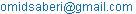(O. Saberi)

*Corresponding author

Omid Saberi, Majid Galoie. Numerical Modeling of Flow Around Groynes with Different Shapes Using TELEMAC-3D Software. American Journal of Water Science and Engineering. Vol. 2, No. 6, 2016, pp. 1-10. doi: 10.11648/j.ajwse.20160206.11

Received: January 4, 2017; Accepted: January 18, 2017; Published: February 16, 2017

Abstract: This paper provides numerical simulation of flow around a Groyne and models the ratio of the reattachment length, water depth and velocity on the surface of the channel and the shear stress on the bottom of the channel between the different types of Groynes in the same hydraulic condition for all models. Moreover, a comparison between the results have been done and the most efficient type of Groynes which have more influence on the hydraulic parameters is chosen. The open source TELEMAC-3D software is used for this numerical modeling and k-ε model was selected for turbulence modeling of the flow simulation. For validation test, our results are compared against three-dimensional computational flow model around a Groyne by Ouillon and Dartus  and experimental result of Holtz . This paper provides a good modeling tools to choose the optimum shape and size for Groynes in rivers for bank protection and river stream training.

Keywords: Different Groyne Shapes, TELEMAC-3D Software, Numerical Modeling, Finite Element Method

Contents

1. Introduction

Groynes are built in different shapes and installed in different parts of a river flow direction. In general, they are used for many purposes in the rivers but the two main reasons for building groynes are the river bank protection and river stream training. For the river bank protection, groynes are installed to divert flow around a river bank to protect them from erosion and for the river stream training, groynes are installed to direct the flow to desirable direction.

Groynes are hydraulic structures with different material, type, shape, size and dimension. Recently, many researches have been done experimental and numerical modeling on groynes and flow around them. Their results show that using different type of groynes in the river has impressive influence on the behavior of the flow in downstream of the river. The most important one which has significantly effect on the water flow and should be considered is the separation zone.

The separation zone (vortex) is the major part of a turbulence flow in the fluid dynamic studies. This zone is usually occurred in parts of fluid with high Reynolds number, and has most rotation in alternating directions. Two alternative methods are normally available for modeling turbulence conditions. First one is the Large Eddy Simulation (LES) and second one is the turbulence modeling, in which, second method solution is based on five approaches called as zero equation models, one equations models, two equations models, Reynolds-stress/flux transport equations models and algebraic stress/flux models. In fluid dynamic studies one of the famous method is the k-ε method (two equations models), the most advantage of this method is stable calculation that easily relative converges and Reasonable predictions fluid fields. Therefore, in this study, the k-ε approach is used for modeling the turbulence of flow. Furthermore, surface velocity, water depth and shear stress around groynes, were calculated. Finally influence of groynes shapes on the turbulence as well as reattachment length for each type of groynes are compared and discussed.

2. Validation

3D Numerical modeling of flow around vertical groynes by Ouillon and Dartus  are used as reference to validate the TELEMAC-3D software. The Ouillon and Dartus used a rectangular channel with 30 m length, vertical smooth wall and the manning coefficient of the bottom of channel was 0.02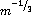, the mean water depth was 0.023 m and the mean velocity was 0.345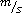as shown in figure 1. The rectangular groyne was used in the middle of this channel with the 25 cm length and 2.5 cm thickness. Sylvian Ouillon and Denis Dartus  did their 3D-numerical modeling according to the experimental modeling of the Holtz . Ouillon and Dartus  used standard k-ε model coupled with the boundary condition suggested by Celik and Rodi  for their numerical modeling. For more information, the reader can refer to the 3D Numerical modeling of flow around vertical groynes by Ouillon and Dartus .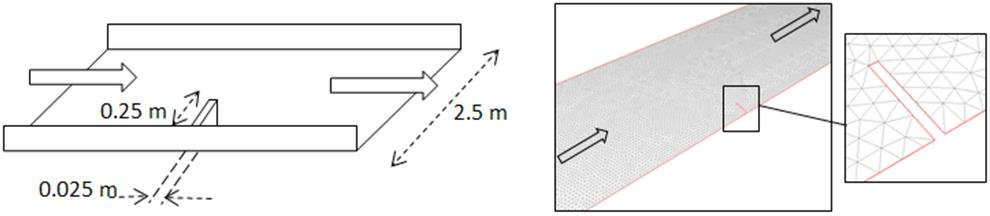Figure 1. Experimental shape of the channel and the TELEMAC-3D numerical modeling.

2.1. TELEMAC-3D

The TELEMAC software has been developed by the Department Laboratorie National d´Hydrauliquie (LNH) at the Electronic de France Direction des Etudes et Recherché (EDF-DER). The software system is owned by EDF-R&D and this software available as the open source software. The TELEMAC system is able to solve shallow water equations, (TELEMAC-2D) and Navier-Stokes Equations (TELEMAC-3D), the basic solution procedure in the TELEMAC-3D system is divided in four steps by solving the Reynolds-averaged Navier-Stokes equations (equations 1 to 4). In the first step, velocity advections is calculated by the momentum equation and in the second step the diffusion and source terms are solved and in the last step, water depth is computed by continuity equation and momentum equation which integrated along vertical direction, for more detail refer to the J.-M. Hervouet .

Continuity equation: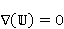(1)

Momentum equation in X- coordinate: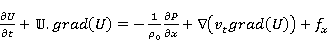(2)

Momentum equation in Y- coordinate: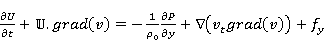(3)

Momentum equation in Z- coordinate: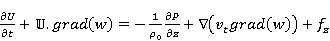(4)

Where P is the pressure [N/m2] and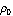is the reference density [kg/m3],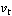is the turbulence viscosity and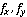are source terms such as the Coriolis force and buoyancy.

U, V, and W are the Cartesian velocity components of the velocity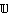[m/s], the friction will appear later as boundary condition of the diffusion terms, with a hydrostatic pressure assumption.

2.2. Numerical Modeling by TELEMAC-3D

In order to model Holtz experimental test by TELEMAC-3D, the mesh of this channel generated by the BLUE KENUUE software. The triangular cells are used in this model. The mesh size in this model vary between 0.04-0.05 m for each side and this size is constant in the whole domain. Also for computing turbulent condition, the k-ε model takes into account for horizontal and vertical scheme. The time step was set to 0.1s, the TELEMAC3D was configured with 4 horizontal levels along the depth, the liquid boundary conditions were the constant discharge for the inlet and the constant height for the outlet of the channel. The non-hydrostatic version of TELEMAC-3D was used. Amount of the manning coefficient was 0.02for the bottom and the smooth wall with the no friction was selected for the lateral boundary conditions.

This channel was modeled for different scheme of velocity advection, turbulent model advection and depth advection, turbulence model advection and depth advection. The results have been shown that the best scheme for velocity advection and turbulence advection was explicit scheme + MURD and the best scheme for depth advections was explicit scheme + MURD PSI.

2.3. Validation

2.3.1. Water Depth

Figure 2 shows the comparison between three results of the experimental model (Holtz ), the numerical modeling Ouillon and Dartus  and the result of the TELEMAC-3D modeling. This Figure clearly shows that TELEMAC-3D result is very close to the experimental result, but it slightly overestimate iso-lines related to 0.233 m water height.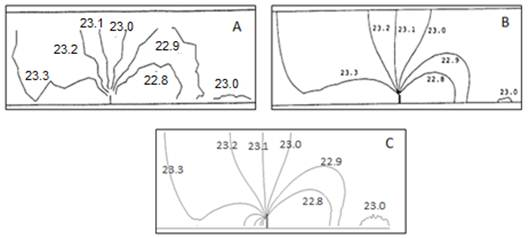A) Exprimental result, Holtz 1991 B) Numerical result, Ouillon and Dartus  C) Result of the TELEMAC-3D

Figure 2. Water depth in the channel.

2.3.2. Velocity Profile

The velocity profiles at Z=0.17 m above the bottom of the channel have depicted in Figure 3 which shows the TELEMAC-3D result is so close to the experimental result.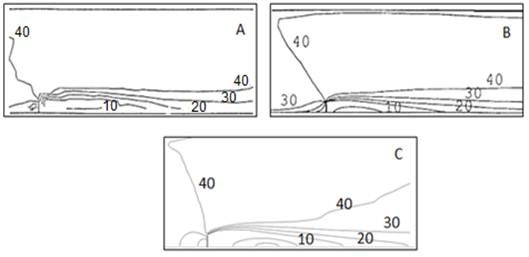A) Exprimental result, Holtz  B) Numerical result, Ouillon and Dartus  C) Result of the TELEMAC-3D

Figure 3. Velocity at the 0.17 m above the bottom of the channel.

2.3.3. Reattachment Length

The reattachment length is the dimensionless parameter and defined as the ratio of the flow separation length to the groyne length. The separation length is defined as the distance from downstream side of the groyne to the zone that velocity becomes zero. In this test the reattachment length was calculated by the TELEMAC-3D, is 11.54 and the amount of the reattachment length was modeled by Ouillon and Dartus , is 10.7 and the experimental value, was obtained by Holtz  is 11.5 which demonstrate that the result from TELEMAC-3D modeling is very close to the experimental result. The final results are shown in the Table 1.

Table 1. Compare the reattachment length modeled by TELEMAC-3D with Experimental results

 Test Name Holtz Result Experimental TELEMAC-3D Result Ouillon and Dartus Result Reattachment length (m) 11.5 11.54 10.7

3. A New Numerical Modeling with TELEMAC-3D

The comparisons between the TELEMAC-3D results and the experimental results in the previous part show that the result of the TELEMAC software was very close to the results of the experimental models.

Now, we want to define new numerical models that show the influence of different shape of groynes on the flow parameters like water velocity and water depths. For this purpose, different types of groynes are divided into two groups. The first group contains groynes with the flanges where length of these flanges varies in the different size and direction while the second group contains groyne without flanges but the width of groynes changes in the different size. Totally 10 different types of groynes were modeled. Figure 4 shows detail of these modelled groynes which can be classified into five different groups as T-shape, L-shape, rectangular, triangular and trapezoidal. In T-shape group only the flange of the T-shape is changed, in L-shape group the size and direction (flanges in the right direction or flanges in the left directions) of flange is differs while in the rest only one type for each group will be considered. In the following, we will model the effect of these different shapes on water velocity and water depth and results will be presented in figures 5-10.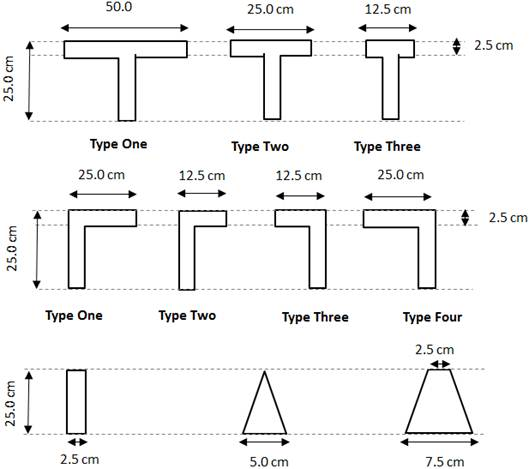Figure 4. Different type of the T-shape, L-shape and rectangular, triangular and trapezoidal shape.

4. Result of Numerical Modeling with TELEMAC-3D

4.1. Water Surface Velocity

4.1.1. T-shape Groynes

Figure 5 shows results of water velocity on the surface around the T-shapes groynes which are modeled by the TELEMAC-3D software. These results indicate that by increasing the length of the flange surface velocity decreases, and the place of this high velocity is located on the left side of the flange. However, reducing the length of the flange causes the surface velocity is increase in the separation zone near the wall in down side of the groyne. Moreover, it can be seen in this figure that by increasing the length of the flange in the upside of the groynes, surface velocity reduce behind the flanges.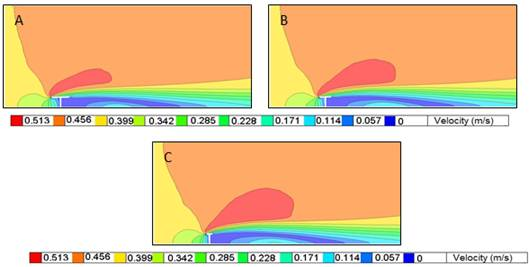A) Type one B) Type two and C) Type three

Figure 5. Surface velocity profile in the different T-shape of the groynes.

4.1.2. L-shape Groynes

Figure 6 shows results of TELEMAC-3D software for the L-shape groynes. In the L shapes groynes totally we have two different results, in the first result by increasing the length of the flanges in downstream of the groynes (figure 6-A and figure 6-B), the surface velocity profile is slightly increased in the separation zones near the walls. But in the second results when the flanges of the groynes are located in the right direction (figure 6-C and figure 6-D), the velocity profile increased in separation zones when the length of the groynes becomes shorter.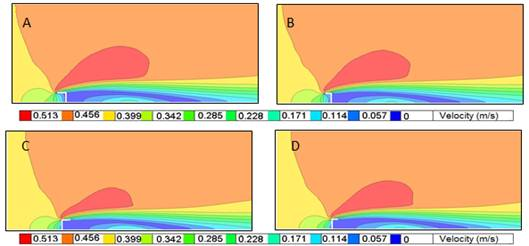A) Type one B) Type two and C) Type three D) Type four

Figure 6. Surface velocity profile for different L-shape types of the groynes.

4.1.3. Rectangular Shape, Trapezoidal Shape and Triangular Shape Groynes

Figure 7 shows the area of the highest surface velocity (red area) within the channel when the rectangular, trapezoidal and triangular groynes shapes are used. This figure indicate that the triangular groyne shape make the high surface velocity occur in the less area compared with the other two types of the groynes and also less velocity will be expected in the separation zone when using this shape type groyne. It can also be seen that in the channel with the rectangular shape we have maximum velocity area compared with two other types. Moreover in the trapezoidal groyne shape the high surface velocity occurred in separation zone near the wall in downstream of the groyne.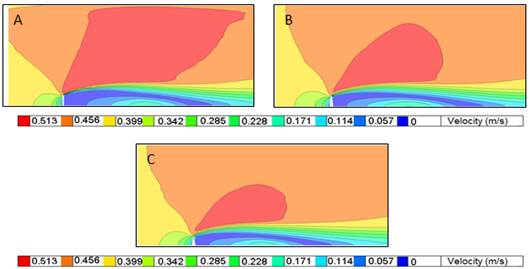A) The rectangular B) the trapezoidal C) The triangular groyne shape

Figure 7. Surface velocity profile in different shapes of groynes.

4.1.4. Reattachment Length

In the table 2, the separation zone length and reattachment length were calculated. Comparison between the mentioned results show that in the T-shape groynes, by increasing length of the flange, the separation zone length decreased also the maximum values of the separation length and reattachment length occurs in the rectangular groyne shape.

Table 2. The vortex length and the reattachment length for all types of groynes.

 Groyne shape Type separation zone length (m) reattachment length T-shape ONE 2.190 (min) 8.76 (min) T-shape TWO 2.397 9.588 T-shape THREE 2.528 10.112 L-shape ONE 2.525 10.1 L-shape TWO 2.408 9.632 L-shape THREE 2.451 9.804 L-shape FOUR 2.547 10.188 Rectangular shape - 2.886 (max) 11.544 (max) Trapezoidal shape - 2.863 11.452 Triangular shape - 2.567 10.268

4.2. Water Depth

4.2.1. T-shape Groynes

Figure 8 shows the T-shape groynes effects on water depth. It shows that by increasing the length of the flange, the depth of the water is increased in downstream of the groynes.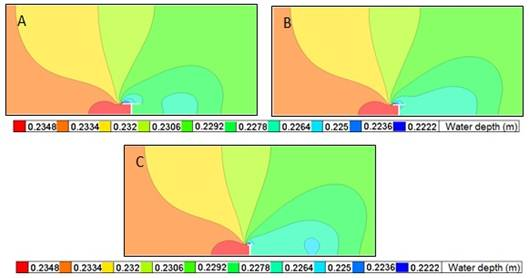A) Type one B) Type two and C) Type three

Figure 8. Water depth profile T-shape groynes.

4.2.2. L-shape Groynes

Figure 9 is a comparison between the same results for L-shape groyne. It shows that in the type three of the L-shape groyne, water depth increased in the downstream of the groyne compared to the other types of the L-shapes groynes.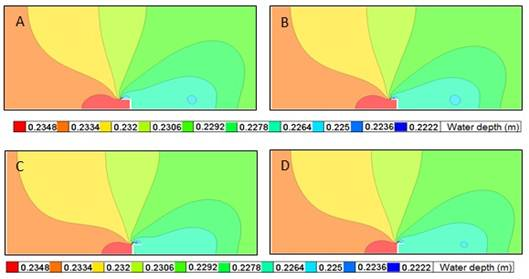A) Type one B) Type two and C) Type three D) Type four

Figure 9. Water depth in different L-shape types of the groynes.

4.2.3. Rectangular Shape, Trapezoidal Shape and Triangular Shape Groynes

According to the figure 10, the water depth area after the downstream side of the groyne is increased in the channel with the Triangular groyne.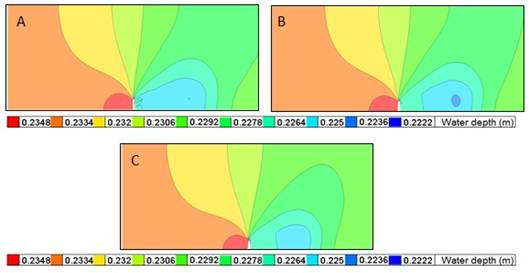A) The rectangular B) the trapezoidal C) The triangular groyne shape

Figure 10. Water depth in the rectangular.

5. Shear Stress on the Bed of the Channel

Water flow applies forces on the bed of the channel which is called as shear force (). These force depends on height of water (h), water velocity (u) and roughness of the bed. In the theoretical consideration the following steps are used to calculate bed shear stress:

In the first step the friction slope is assumed equal to the bottom slope.

In the second step logarithmic distribution is assumed for velocity distribution near the walls (Prandtl  and Von Karman ), as shown in the equation 5, 6 and 7.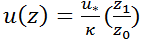(5)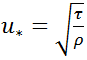(6)

Where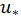is the shear velocity,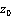is expressed as a function of the Nikuradse bed roughness (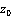= ks/30), with ks is the Grain roughness height,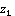is the distance of the ﬁrst vertical plane from the bed level [m] and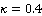is the von Karman constant.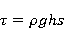(7)

Where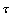is the bed shear stress [N/m2],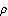is the density of water [kg/m3], h is the water depth [m], s is the bottom slope and g is the Gravitational acceleration [m/s2].

In this paper for calculating bed shear stress the SYSIPHE software is used.

5.1. SISYPHE Software

In order to model erosion process and sediment transport in river the SISYPHE software has been developed in the TELEMAC-MASCARET system. The SISYPHE software can simulate sediment transport and bed elevation in the complex morphology same as coastal, rivers, lakes and estuaries with the different discharge rate, different sediment grain size and different sediment transport equations. The SISYPHE software can be easily coupled with the TELEMAC-2D and TELEMAC-3D software. In this coupling, at each time step TELEMAC-2D or 3D send calculated Hydrodynamic parameters like height of the water (H) and water velocity (U, V) to the SISYPHE software.

The SISYPHE software model bed elevation by solving the two-dimensional sediment continuity equation which is called the Exner equation as shown in the Equation 8 (Sisyphe):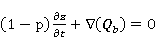(8)

Here,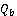is the bed load transport [m3/s], z is the bed elevation [m] and p is the bed porosity.

5.2. T-shape Groynes

Figure 11 shows that by decreasing the length of the flange in the T-shape groynes, shear stress on the bottom of the channel near the left side of the flange is increased. It also shows the area of the erosion in the short T-shape groyne (type three) is increased. The rate of the erosion in downstream of the groyne, near the wall was also decreased by increasing the length of the flanges in all types of the T-shape groynes.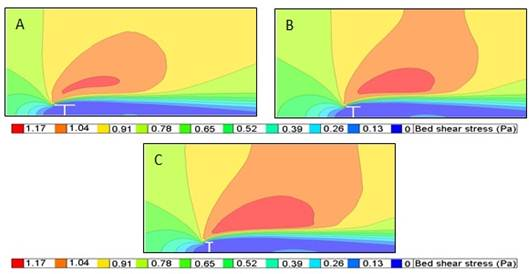A) Type one B) Type two and C) Type three

Figure 11. Bottom shear stress in the different T-shape of the groynes.

5.3. L-shape Groynes

Figure 12 shows effects of L-shape groynes on the bottom shear stress changes. Figure 12-A and 13-B shows that by increasing the flange length, shear stress decreased on the bed of the channel but in the Figure 12-C and 12-D amount of the shear stress increased when the length of the flange increasing. Also according to the figure 12 the maximum shear stress in the separation zone happened in type C and D.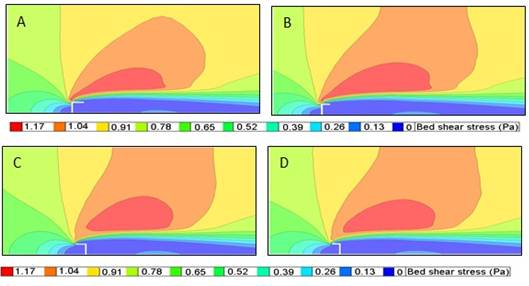A) Type one B) Type two and C) Type three D) Type four

Figure 12. Bottom shear stress in different L-shape types of the groynes.

5.4. Rectangular Shape, Trapezoidal Shape and Triangular Shape Groynes

Figure 13 shows the modeling results for the rectangular, trapezoidal and triangular gryones on the bottom shear stress. It shows that the rectangular shape causes maximum erosion and shear stress on the bed of the channel. The maximum erosion near the wall in the downstream of the groynes occurred in the trapezoidal groyne shape. However, rectangular shape makes the maximum shear stress in the upside of the groynes near the wall. Also triangular shape make less shear stress and erosion in the bed and bank of the channel compared with other three types.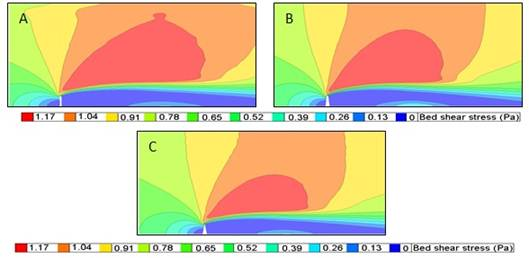A) The rectangular B) The trapezoidal C) The triangular groyne shape

Figure 13. Bottom shear stress in the rectangular.

6. Conclusion

This study has been solved the Reynolds-averaged Navier-Stokes equations with the finite elements method to simulate flow in the experimental channel and also for simulating turbulent flow in the channel the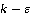model was used. The results of the simulation are validated against original model (the Holtz ).

Furthermore, the results of this simulation are used to investigate effects of different groynes shapes (10 different types) on the flow characteristics within the understudied channel. The effects of different groynes shapes on flow characteristics within the channel can be summarized as below:

The results from the groynes without flanges (rectangular, trapezoidal and triangular shapes) show that these types create the maximum shear stress on the bottom. It means that maximum scoring will be happened around the groynes, on the right sides of channel and within the separation zone. This also indicates that more shear stress in the bank of the channel can occur using these types of groynes inside a channel. Other results regarding this shape type groyne are:

The groynes without flanges cause the maximum surface velocity.

In the groynes without flanges the rectangular shape has the maximum erosion in the bottom of the channel

In the groynes without flanges the trapezoidal shape has the maximum erosion in the bank of the channel.

In the groynes without flanges the rectangular shape has the maximum reattachment lengths compared to the other types of the groynes.

The groynes without flanges have maximum water depth in upstream side of the groynes in the channel compared to the other types of the groynes.

The length of the flanges in the groynes has direct influence on the bottom shear stress and the shear stress in the separation zone. Other results regarding this shape type groyne are:

In the T-shape groynes, increasing length of the flange make the velocity in surface decreased. It also decreased the shear stress on the bottom and decreased the shear stress on the bank of the channel.

Increasing the length of the flange make velocity to decrease behind flanges.

In the T-shape groynes, increasing the length of the flange, decreasing the reattachment length.

In the L-shape groynes, if the flanges installed in the opposite flow directions, by increasing length of the flange, the shear stress increased on the bottom and on the bank of the channel, and if the flanges installed in the flow directions, by increasing length of the flange, the shear stress decreased on the bottom and on the bank of the channel.

The groynes with the flanges, in downstream side of the groynes the depth of the water has the maximum value compared to the other types of the groynes.

Totally It can be said that:

The groynes with the flange exert less erosion around the groyne on the bottom of the channel and on the bank of the channel in comparison to the groynes without flanges.

The groynes without the flange increased the depth of the water in upstream side of the groyne in the channel in comparison to the groynes with flanges.

References

1. Athanasios N. Papanicolaou,John T. Sanford,Dimitrios C. Dermisisand and Gabriel A. Mancilla. (2010). A 1-D morphodynamic model for rill erosion. WATER RESOURCES RESEARCH.
2. Azinfar, H. (2010). Flow Resistance and Associated Backwater Effect Due To Spur Dikes In Open Channel. University of Saskatchewan, P.h.D Thesis.
3. C. B. Vreugdenhil and J. H. A. Wijbenga. (1982). COMPUTATION OF FLOW PATTERNS IN RiVERS. ASCE, 87-90.
4. Celik, I. and Rodi, W. (1991). "Suspended sediment-transport capacity for open channel flow." Journal of Hydraulic Engineering, ASCE, 117(2), 191-204.
5. Holtz, K. P. (1991). Numerical simulation of recirculating flow at groynes, Computer Methods in Water Resources, 2(2), 463-477.
6. H. Tennekes and J. L. Lumley. (1972). A first course in turbulence. 6th Edition, MIT Press.
7. H. Weilbeer and J. A. Jankowski. (2000). A Three–Dimensional Non–Hydrostatic Model for Free Surface Flows. ASCE, 162-177.
8. J.-M. Hervouet and E. Razafindrakoto. (2005). The wave equation applied to the solution of Navier-Stokes equations in finite elements. U.K: WIT press.
9. M. R. KABIR and NASIR AHMAD. (1996). Bed Shear Stress for Sediment Transport in The River JAMUNA. Journal of civil engineering.
10. Ouillon, S and Dartus, D. (1997). Three-dimensional computation of flow around groyne.Journal of Hydraulic Engineering, 123(11), 962-970
11. PRANDTL, L. 1925 Bericht uber untersuchungen zur ausgebildeten turbulenz.Z. Angew. Math. Mech.5, 136–139..
12. Sisyphe v6.3 User's Manual. (January 2014).
13. Schmock,L.r;Hager,W. (2012).Plane dike-breach due to overtopping: effects of sediment, dike height and.IAHR, 576-587.
14. TELEMAC-3D Software User's Manual, Release 6.2.(March 2013).
15. Von karman, T. 1930 Mechanische ahnlichkeit und turbulenz. ¨ Gott. Nachr. 58–76.

 Contents 1. 2. 2.1. 2.2. 2.3. 3. 4. 4.1. 4.2. 5. 5.1. 5.2. 5.3. 5.4. 6.
Article ToolsAbstractPDF(471K)